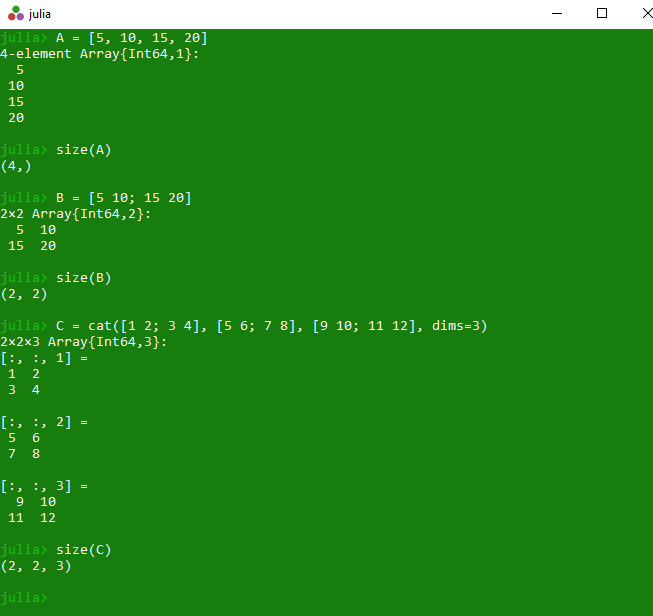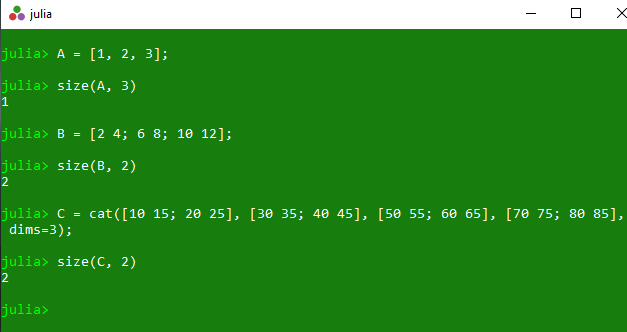# Get array dimensions and size of a dimension in Julia – size() Method

The `size()` is an inbuilt function in julia which is used to return a tuple containing the dimensions of the specified array. This returned tuple format is (a, b, c) where a is the rows, b is the columns and c is the height of the array.

Syntax:
size(A::AbstractArray)
or
size(A::AbstractArray, Dim)

Parameters:

• A: Specified array
• Dim: Specified dimension

Returns: It returns a tuple containing the dimensions of the specified array.

Example 1:

 `# Julia program to illustrate  ` `# the use of Array size() method ` ` `  `# Finding a tuple containing the dimension of ` `# the specified 1D array A. ` `A ``=` `[``5``, ``10``, ``15``, ``20``] ` `println(size(A)) ` ` `  `# Finding a tuple containing the dimension of ` `# the specified 2D array B of size 2*2 ` `B ``=` `[``5` `10``; ``15` `20``] ` `println(size(B)) ` ` `  `# Finding a tuple containing the dimension of ` `# the specified 3D array C of size 2*2*2 ` `C ``=` `cat([``1` `2``; ``3` `4``], [``5` `6``; ``7` `8``], [``9` `10``; ``11` `12``], dims``=``3``) ` `println(size(C)) `

Output:Example 2:

 `# Julia program to illustrate  ` `# the use of Array size() method ` `    `  `# Finding a tuple containing the dimension of ` `# the specified 1D array A. ` `A ``=` `[``1``, ``2``, ``3``]; ` `println(size(A, ``3``)) ` `    `  `# Finding a tuple containing the dimension of ` `# the specified 2D array B of size 3*2 ` `B ``=` `[``2` `4``; ``6` `8``; ``10` `12``]; ` `println(size(B, ``2``)) ` `    `  `# Finding a tuple containing the dimension of ` `# the specified 3D array C of size 2*2*4 ` `C ``=` `cat([``10` `15``; ``20` `25``], [``30` `35``; ``40` `45``], ` `        ``[``50` `55``; ``60` `65``], [``70` `75``; ``80` `85``], dims``=``3``); ` `println(size(C, ``2``)) `

Output:My Personal Notes arrow_drop_upCheck out this Author's contributed articles.

If you like GeeksforGeeks and would like to contribute, you can also write an article using contribute.geeksforgeeks.org or mail your article to contribute@geeksforgeeks.org. See your article appearing on the GeeksforGeeks main page and help other Geeks.

Please Improve this article if you find anything incorrect by clicking on the "Improve Article" button below.

Article Tags :

Be the First to upvote.

Please write to us at contribute@geeksforgeeks.org to report any issue with the above content.